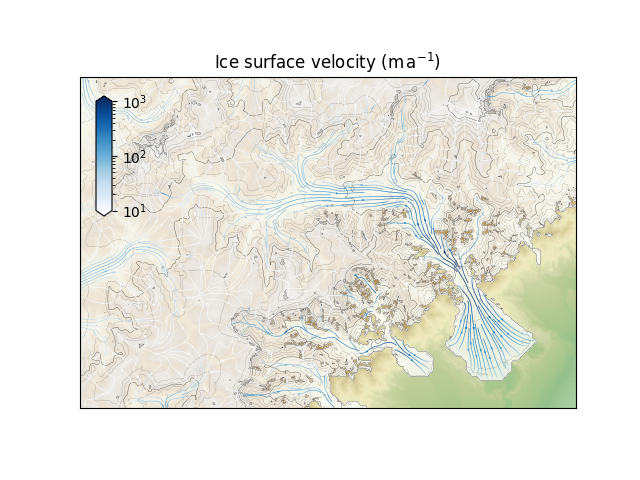# Interpolated streamplot#

Demonstrate interpolating two-dimensional model output onto a higher-resolution topography provided in a separate file. Bedrock isostatic adjustment needs to be informed in order to correct for the offset between the model and the high-resolution bedrock topographies. This is a rather extreme example with a ten-fold increase in horizontal resolution.```import matplotlib.pyplot as plt
import hyoga

# initialize figure
ax = plt.subplot()
cax = plt.axes([0.15, 0.55, 0.025, 0.25])

# open demo data
with hyoga.open.example('pism.alps.out.2d.nc') as ds:

# compute isostatic adjustment from a reference input topography
ds = ds.hyoga.assign_isostasy(hyoga.open.example('pism.alps.in.boot.nc'))

# perform the actual interpolation
ds = ds.hyoga.interp(hyoga.open.example('pism.alps.vis.refined.nc'))

# plot model output
ds.hyoga.plot.bedrock_altitude(ax=ax, cmap='Topographic', center=False)
ds.hyoga.plot.surface_altitude_contours(ax=ax)
ds.hyoga.plot.ice_margin(ax=ax, facecolor='w')

# plot streamplot
streams = ds.hyoga.plot.surface_velocity_streamplot(
ax=ax, cmap='Blues', vmin=1e1, vmax=1e3, density=(6, 4))